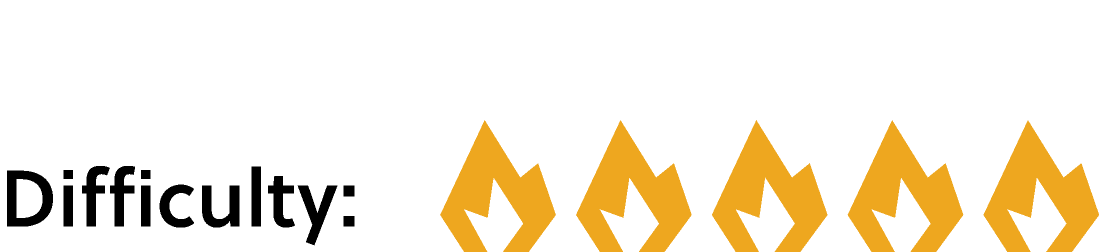## Which Square to Subtract?

For most games, identifying the perfect move is too difficult to do in practice. Chess, for example, has too many possible moves for even the fastest computer to solve it completely (so far), but many chess programs do have the ability to play the game perfectly when there are only a few pieces left on the board.

Today's problem involves a game, and it just might be possible that this game can be solved perfectly. Keep reading to see some examples of how to approach games like this, or jump ahead straight to today's challenge.

One mathematical game is called Nim. It works like this:

• There are several heaps, each containing several stones.
• Taking turns, each player must remove one or more stones from a single heap.
• The last player to remove a stone loses.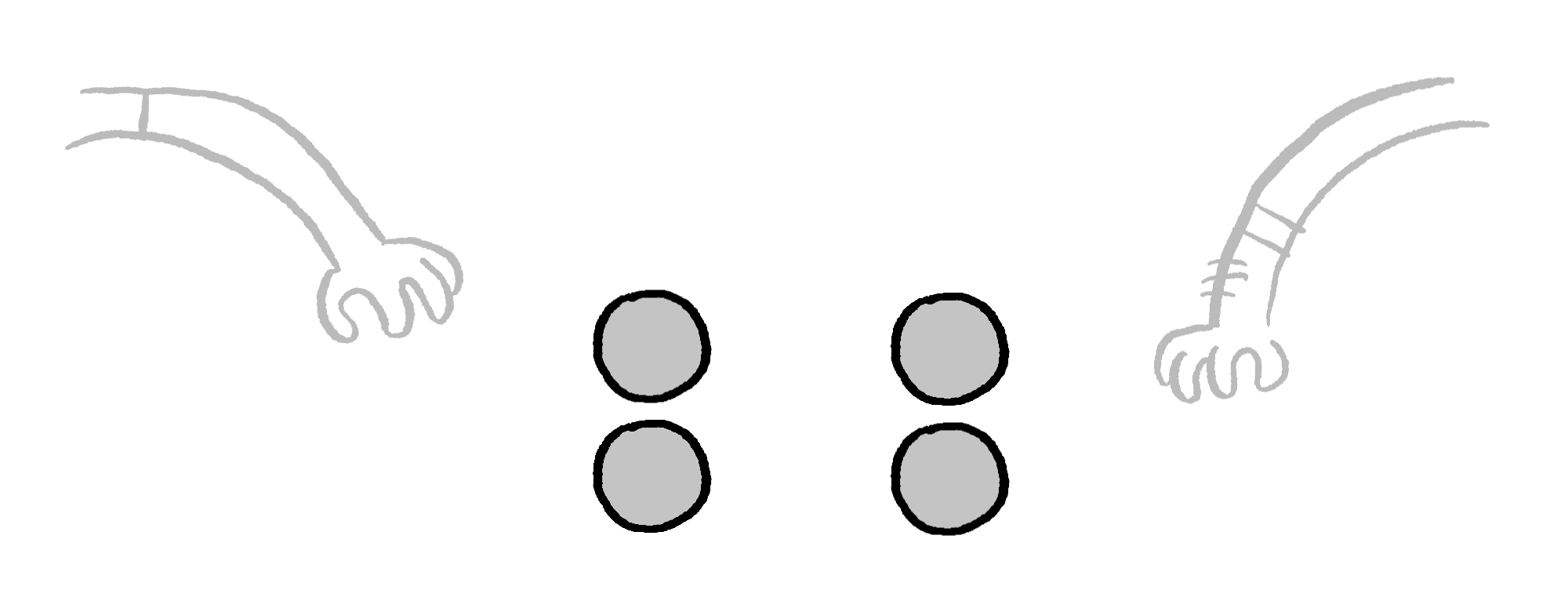Let's look at a very simple version of Nim: two heaps, each with $2$ stones. What are the possible first moves? Since the piles are equal, we can treat taking stones from the left and right as if they are the same move.

Let's look at one possible first move. Try removing just a single stone.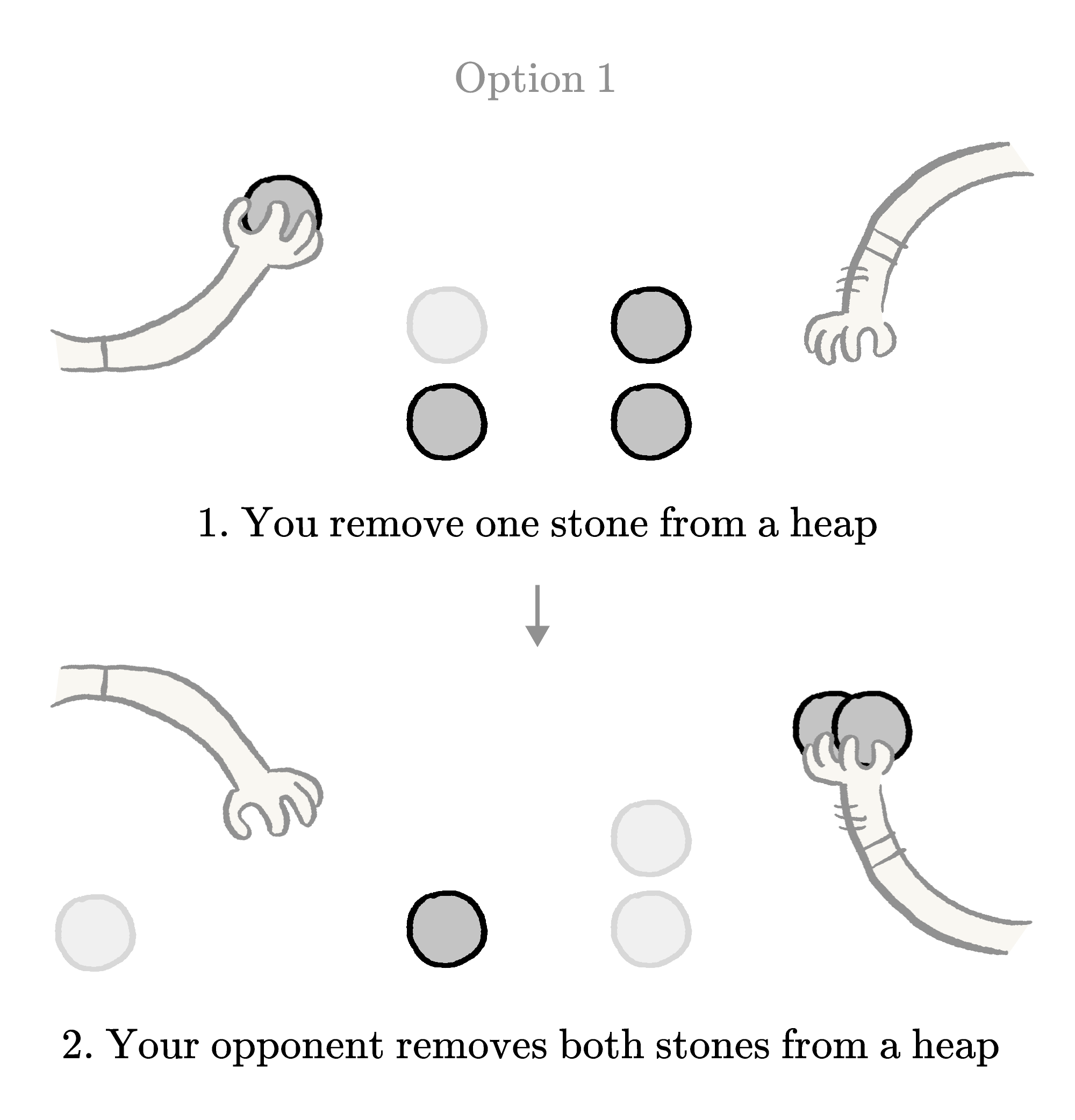If you do that, your opponent can pick up two stones, leaving you with the last stone. That's not good. Let's try a different move: let's remove both stones from a heap on the first move.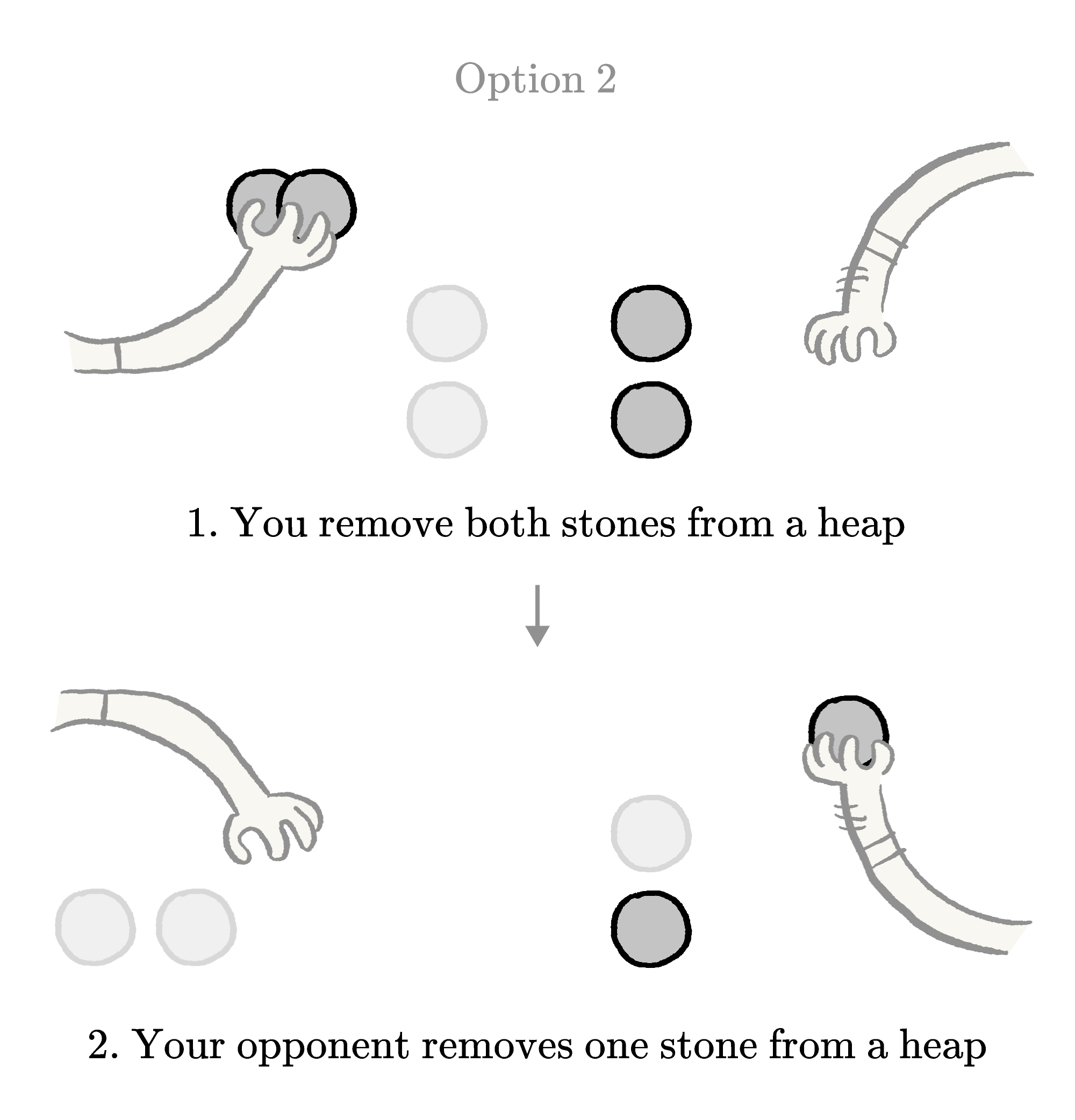Uh oh. This move also makes it easy for your opponent to win. There's no good option for the first move!

If you want to win a game of Nim that starts with two heaps of two stones each, the best move is to make your opponent go first! No matter what they do, you will be able to win.

Let's look at another mathematical game: "$21.$" It's like Nim in some ways, and different in others. The rules are as follows:

• The first player must say "$1.$"
• After that, players take turns adding to the number. They may add any value from $1$ to $3.$
• The first player to say or exceed $21$ loses.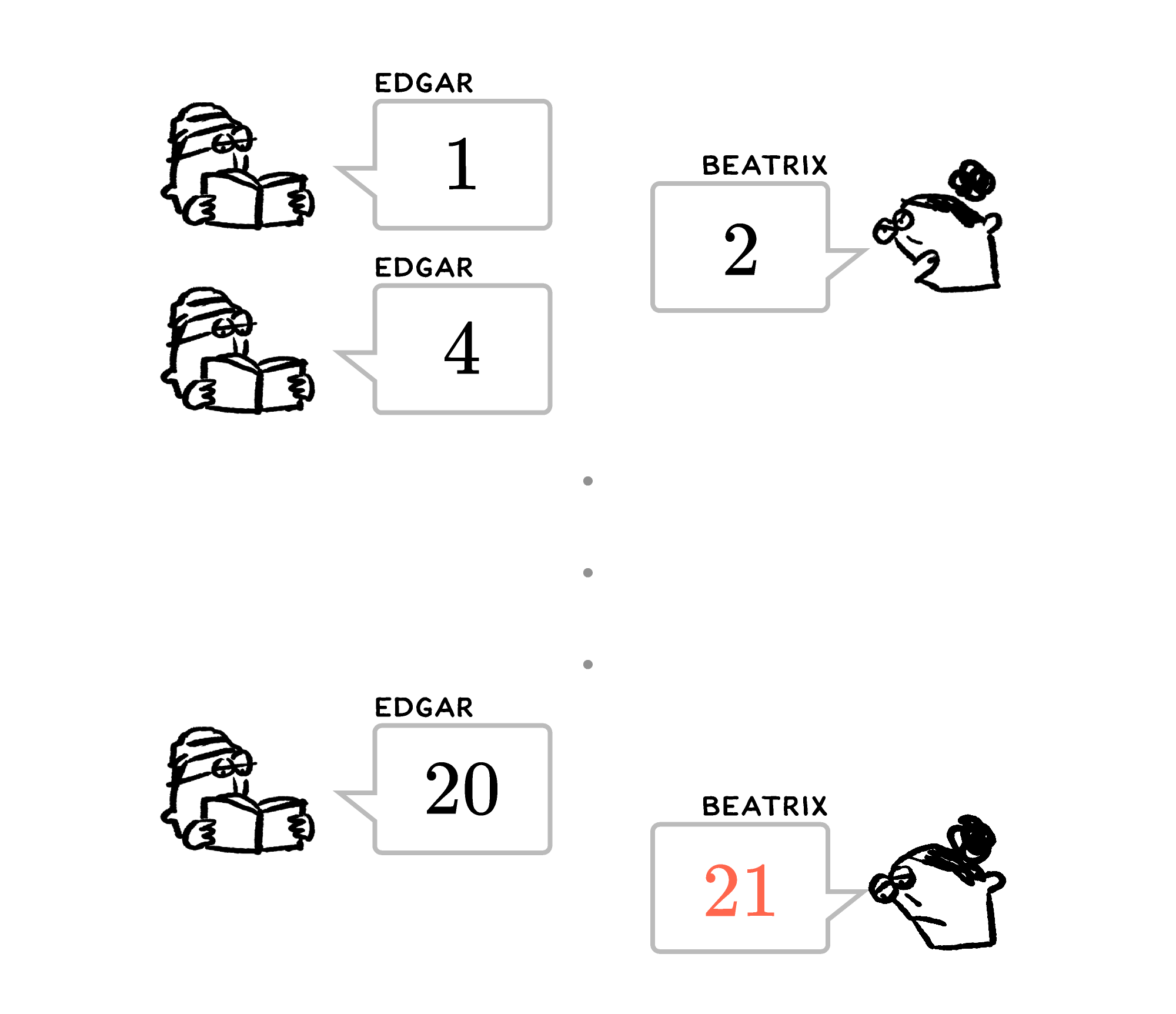What is the optimal strategy for this game? Who will win if both players play perfectly?

One approach that can be helpful for thinking about problems like this is to start from the end. What is the winning move in this game? In this game, if someone says $20,$ then their opponent is forced to say $21$ and lose, so getting to be the one who says $20$ is a winning move. How can we get there?

If we say $17, 18,$ or $19,$ then our opponent will have the opportunity to say $20$ and we will lose, but if we say $16,$ then no matter what our opponent says, we'll get to say $20$ next. Since $20$ is a winning move, so is $16.$

In fact, we can continue to reason our way backwards from here: $16, 12, 8,$ then $4.$ If the second player picks $4$ as their first move and then chooses multiples of $4$ through the entire game, they are guaranteed to win.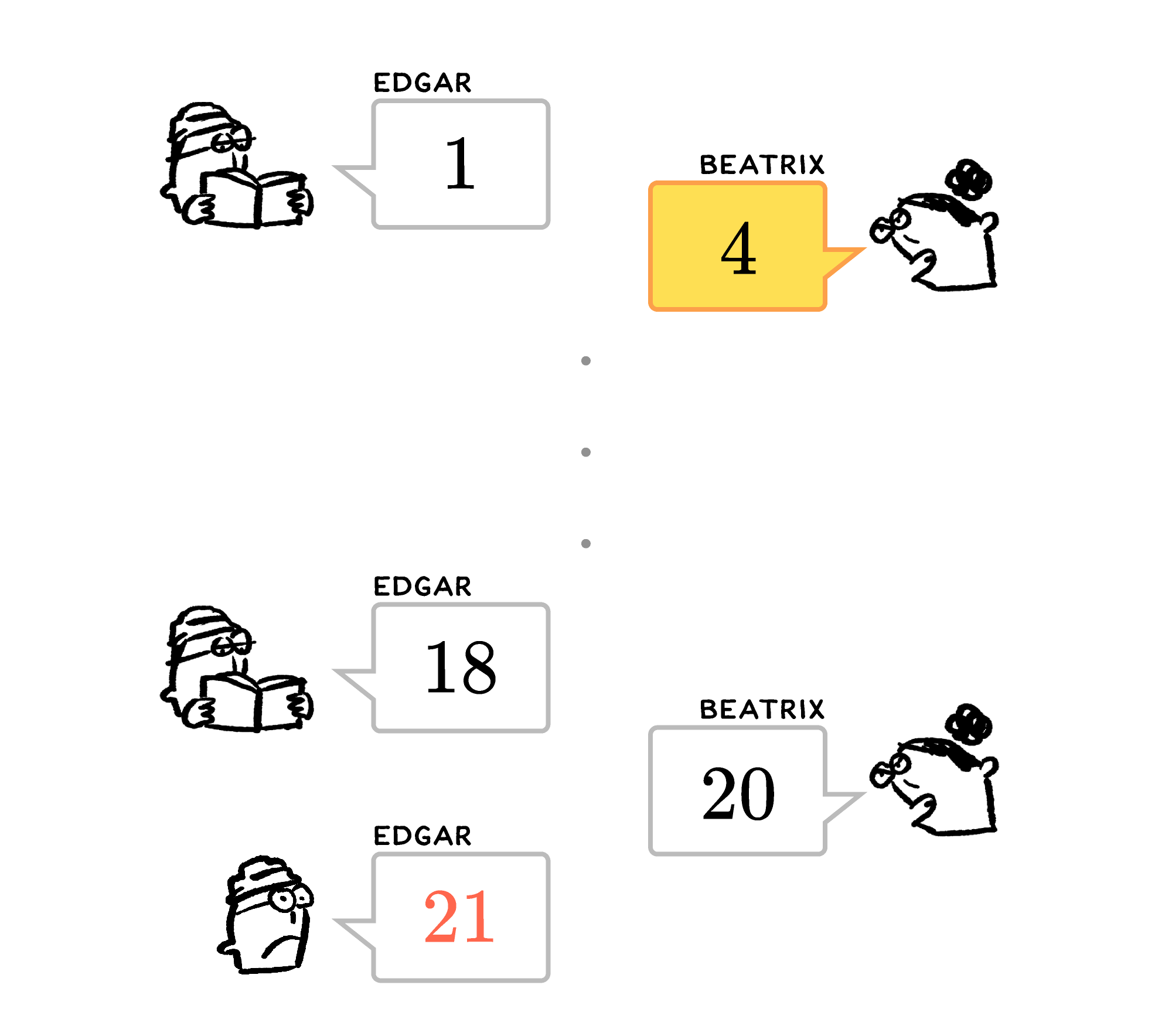Can you use careful reasoning to solve the game below?

# Today's Challenge

Mei and Yuri are playing a subtraction game, with the following rules:

• Together, they select a positive integer $N.$
• The first player subtracts a square number from $N.$ (The result cannot be negative.)
• After that, the players take turns subtracting square numbers from the result of the previous turn's subtraction. (The result cannot be negative.)
• The player who subtracts a square number to make $0$ wins.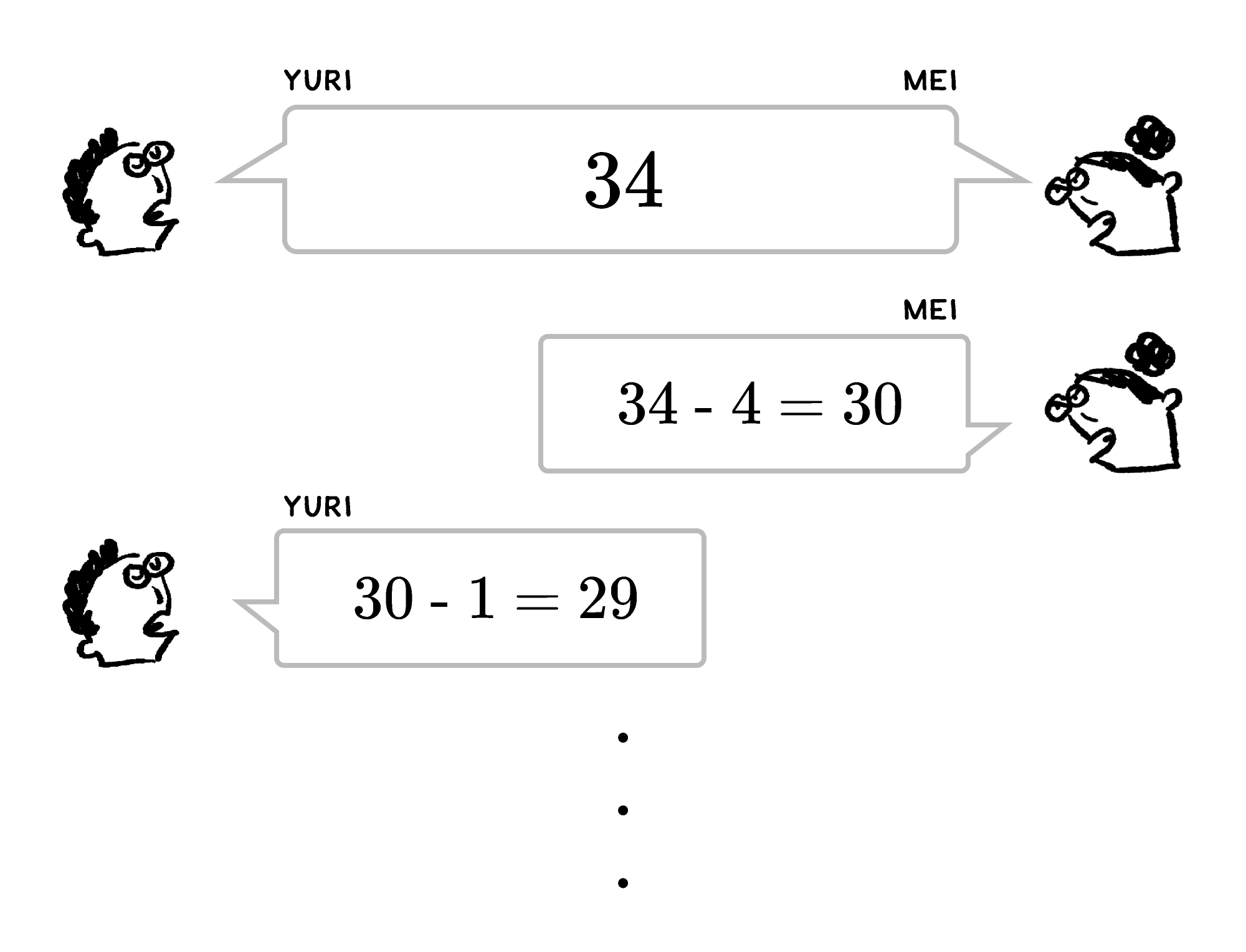This is an example of one way this game could be played.

Suppose Mei and Yuri decide to start with $34,$ and Mei goes first. Assuming both players play perfectly, which number should Mei subtract on her first turn in order to win?